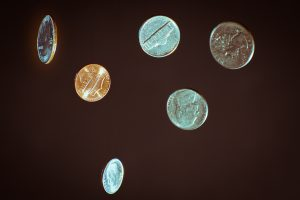# A Bet You Might Not Want To Take – Is A Coin Toss Ever Fair?

Fields covered: Statistics, Probability, Physics

Related Articles:
§How random is the toss of a coin? §Probability of a tossed coin landing on edge |

It shouldn’t matter right? After all, the probability of either appearing should be half, it is entirely luck based. You would be correct theoretically, except well, it’s never half in reality.

Let’s start off by saying that there are in fact more than two outcomes. It is entirely possible for the coin to land on the edge, albeit the probability of that happening being as low as 1 in 6000 tosses.

Even if you were to treat such an outcome as negligible due to its low occurrence relative to the other two outcomes, the odds are still not equal between heads and tails. Depending on the origin of the coin, the outcome may be slightly skewed towards one side due to an uneven centre of mass. Say for instance, your friend is flipping a Washington quarter, the design of heads makes it such that it ends up being slightly heavier than tails. As a result, the probability of flipping a head would roughly be 0.51.

Then you might wonder, surely the odds would be equal if it were a fair coin?

Unfortunately, it may not be as equal as you might think. In an experiment conducted, it has been found that given enough time and attempts, one can manipulate the toss of a coin such that the odds deviate significantly from the expected 50-50. In fact, one participant managed to achieve a 0.68 proportion of heads relative to tails when attempting to achieve as many heads results out of 300 tosses.

What does this tell us?

A coin toss may just be about probability theoretically, but in reality, a coin toss only seems to be 50-50 due to the lack of information made known to us. As seen above, just knowing the mass distribution of the coin results in a 2% difference in probability of outcomes. What if we know the initial velocity when the coin leaves the hand, or the angle at which the coin is thrown, or even the height at which your friend is going to catch the coin? The more variables we are able to control, the more likely it is for us to attain the result we want.

So, the real question is, is there true randomness at play in a coin toss? And will you ever be able to guarantee the outcome given all possible information? Although, one thing is for sure, a coin toss should never be something you would want to bet on.

## Other Briefs## Dwellers of the Deepest Deep: A Biochemical Manual to Surviving the Abyss## Laser: Light that Burns## A Bet You Might Not Want To Take – Is A Coin Toss Ever Fair?